Midwest Theory Day

Session 1

9:30-10:15 Konstantin Makarychev (IBM Research), “Vertex Sparsifiers and Lipschitz Extendability”

We study vertex cut and flow sparsifiers that were recently introduced by Moitra, and Leighton and Moitra. We improve and generalize their results. We give a new polynomial-time algorithm for constructing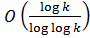cut and flow sparsifiers, matching the best known existential upper bound on the quality of a sparsifier, and improving the previous algorithmic upper bound of O(log^2 k / log log k). We show that flow sparsifiers can be obtained from linear operators approximating minimum metric extensions. We introduce the notion of (linear) metric extension operators, prove that they exist, and give an exact polynomial-time algorithm for finding optimal operators.

We then establish a direct connection between flow and cut sparsifiers and Lipschitz extendability of maps in Banach spaces, a notion studied in functional analysis since 1950s. Using this connection, we obtain a lower bound of Omega(sqrt{log k / log log k}) for flow sparsifiers and a lower bound of Omega(sqrt{log k} / loglog k) for cut sparsifiers. We show that if a certain open question posed by Ball in 1992 has a positive answer, then there exist ~O(sqrt{log k}) cut sparsifiers. On the other hand, any lower bound on cut sparsifiers better than Omega(sqrt{log k}) would imply a negative answer to this question.

This is a joint work with Yury Makarychev (Toyota Technological Institute at Chicago).

10:15-11:00   Chandra Chekuri (UIUC), “Dependent Randomized Rounding for Matroids and Applications”

We describe algorithms for randomly rounding a fractional solution in a matroid (base) polytope to an integral one. We present the swap rounding technique which clarifies the role of exchange properties of combinatorial structures in dependent rounding schemes. Our main technical results are concentration bounds for functions of random variables arising from these rounding techniques. We prove Chernoff-type concentration bounds for linear functions of random variables and also an exponential lower-tail bound for monotone submodular functions. We will briefly outline the extension of swap rounding scheme for the intersection of two matroids and non-bipartite matching. We will motivate and discuss applications of these rounding schemes.

Joint work with Jan Vondrak and Rico Zenklusen.

11:00-11:20   Gruia Calinescu (IIT), “Minimum Power Strong Connectivity”

Given a directed simple graph G=(V,E) and a nonnegative-valued cost function the power of a vertex u in a directed spanning subgraph H is given by the maximum cost of an arcs of H exiting u. The power of H is the sum of the power of its vertices.

Power Assignment seeks to minimize the power of H while H satisfies some connectivity constraint. In this paper, we assume E is bidirected (for every directed edge e in E, the opposite edge exists and has the same cost), while H is required to be strongly connected. This is the original power assignment problem introduce in 1989 and since then the best known approximation ratio was 2 and is achieved by a bidirected minimum spanning tree.  We improve this to 1.98. We do this by combining techniques from Robins-Zelikovsky (2000), Christofides (1976), and Caragiannis, Flammini, and Moscardelli (2007), together with a novel property on T-joins in strongly connected hypergraphs.

11:20-11:40   Alina Ene (UIUC), “Prize-Collecting Steiner Tree and Forest in Planar Graphs”

In the prize-collecting Steiner tree problem, we are given a graph G with weights on the edges and penalties on the vertices, and the goal is to find a minimum cost tree, where the cost of a tree is the total weight of its edges plus the total penalty of the vertices not in the tree. In the prize-collecting Steiner forest problem, there is a penalty associated with each pair of vertices and the goal is to find a minimum cost forest, where the cost  of a forest is the total weight of its edges plus the total penalty of the pairs not connected by the forest.

In this talk, we present polynomial-time approximation-preserving reductions (up to a factor of 1 + \epsilon) from the prize-collecting Steiner tree and prize-collecting Steiner forest problems in planar graphs to the corresponding problems in graphs of bounded treewidth.

Joint work with Chandra Chekuri and Nitish Korula.

11:40-12:00  Kyle Fox (UIUC), “Online Scheduling on Indentical Machines using SRPT”

It is known that SRPT achieves the best possible competitive ratio for total flow time on identical machines up to a constant factor. Using resource augmentation, SRPT is known to achieve total flow time at most that of the optimal solution when given machines of speed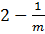. However, a gap has persisted in our understanding of SRPT. Before this work, the performance of SRPT was not known when SRPT is given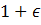-speed when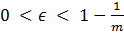, even though it has been thought that SRPT is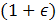-speed O(1)-competitive for over a decade. We show SRPT is-speed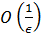-competitive for all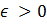.

Session 2

1:00-1:20      Matthew Anderson (University of Wisconsin, Madison), “Derandomizing Polynomial Identity Testing for Multilinear Constant-Read Formulae”

Polynomial identity testing is the fundamental problem of deciding whether a given polynomial identity holds. The problem has a natural and efficient randomized algorithm, but efficient deterministic algorithms for the general case have remained elusive. Indeed, derandomizing the general case would imply circuit lower bounds that have been a central open problem in complexity theory for nearly half a century.

We give the first deterministic polynomial-time algorithm for testing whether multilinear constant-read arithmetic formulae are identically zero. In such a formula each variable occurs only a constant number of times and each subformula computes a multilinear polynomial. We also develop an algorithm that works in a blackbox fashion and runs in quasi-polynomial-time in the number of variables. When the formula also has bounded depth this algorithm can be specialized to run in polynomial-time. Our most general results encompass recent deterministic identity tests for sums of a constant number of read-once formulae, and for multilinear depth-four formulae.

In the talk we will give a high-level overview of our approach, which focuses on a way of exhibiting substructure in constant-read formulae that acts as a witness of non-zeroness.

Joint work with Dieter van Melkebeek and Ilya Volkovich.

1:20-1:40      Balasubramanian Sivan (University of Wisconsin, Madison), “Single-Call Mechanisms”

Following Babaioff, Kleinberg, and Slivkins [EC'10], we study single-call mechanisms —  truthful mechanisms that evaluate an allocation function only once per instantiation. First, we show that single-call mechanisms are possible for maximal-in-distributional-range (MIDR) allocation rules, i.e. computing truthful payments is essentially as easy as computing a single allocation. We give a procedure that transforms a multi-parameter maximal-in-distributional-range (MIDR) allocation rule into a truthful in expectation mechanism that makes a single black-box call to the allocation function. The resulting mechanism gives the optimal outcome with probability arbitrarily close to 1. We also characterize all single-call black-box reductions for MIDR allocation rules and prove our transformation optimizes the trade-off between the probability of selecting the outcome of the original allocation rule and the magnitude of the largest payment. Second, we study optimal transformations in single-parameter settings. Babaioff et al. [EC'10] ask if their transformation optimizes the trade-off between the largest rebate and the probability of selecting the right outcome — we answer this question in the affirmative and show that generalizing their construction gives a family of optimal transformations under two natural definitions of rebate. In the process, we succinctly characterize the space of truthful in expectation single-call transformations for single-parameter domains, and we show that a single-call mechanism may be implemented with no positive transfers if a sufficiently good approximation is known.

We also analyze a special case of the single-parameter setting where the allocation function depends only on the relative order of the bids. We show that a simple construction performs better than Babaioff et al.'s [EC'10] trade-off in this setting.

Joint work with Christopher Wilkens.

1:40-2:00      Eric McDermid (University of Wisconsin, Milwaukee), “Sex-Equal Stable Matchings: Parametrized Complexity and Exact Algorithms”

An instance of the stable marriage problem with incomplete lists (SMI) consists of a set of men and a set of women, each of whom provides a preference list strictly ranking a subset of the members of the opposite set.  The goal is to find a stable matching M — a matching of men to women such that there is no (man,woman) pair not matched to each other who prefer each other over their situation in M.  Every stable marriage instance always admits at least one stable matching, and, in general, the number of stable matchings can be exponential in the size of the input.

In this talk we discuss exact and parameterized computation of a variant of the stable marriage problem in which we seek matchings that are not only stable, but are also “fair” in a formal sense.  In particular, we study the sex-equal stable marriage problem (SESM), in which, roughly speaking, we wish to find a stable matching with the property that the men's overall happiness is as close as possible to the women's overall happiness. This problem is known to be strongly NP-hard.

Motivated by practical applications of this problem, we specifically consider SESM instances in which the preference lists of the men and/or women are bounded in length by a constant. On the negative side, we show that SESM is W-hard, and also inapproximable within a factor of two, even if both the men's and women's preference lists are of length at most three.  This improves upon the previously known NP-hardness results.  On the positive side, we present a low-order exponential-time algorithm for a more general version of SESM.

2:00-2:45      Shuchi Chawla (University of Wisconsin, Madison), “Bayesian Mechanism Design for Budget-Constrained Agents”

Many real-world auction and mechanism design settings involve budget constraints for agents. Specifically, an agent's utility from an outcome is given by his value for the outcome minus any payment he makes to the mechanism, as long as the payment is below his budget, and is negative infinity otherwise. This discontinuity in the utility function presents a significant challenge in mechanism design, and classical "unconstrained" mechanisms fail to work in settings with budgets. In this talk we present new techniques and results for mechanism design with budget constraints in Bayesian settings. This talk is based on joint work with David Malec and Azarakhsh Malekian.

2:45-3:30      Anastasios Sidiropoulos (TTIC), “Discrete differentiation and local rigidity of smooth sets in the plane”

We exhibit an infinite doubling metric space whose n-point subsets require distortion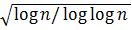to embed into L_1. This nearly matches the upper bound of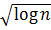(Gupta-Krauthgamer-Lee 2003)and improves over the best previous bound of (log n)^c for some c > 0(Cheeger-Kleiner-Naor 2009). Furthermore, this offers a nearly tight integrality gap for a weak version of the Goemans-Linial SDP for Sparsest Cut, matching the upper bound of Arora-Lee-Naor (2005). We discuss how our results might lead to a resolution of the full Goemans-Linial conjecture.

Following the general approach developed by Cheeger and Kleiner (2006), our lower bound uses a differentiation argument to achieve local control on the cut measure, followed by a classification step of the cuts that can appear. In order to get tight bounds, the classification step has to deal with sets which satisfy only a weak average regularity assumption, meaning that we have to control not just "99%-structured sets," but "1%-structured" sets as well.  This weak regularity is achieved via a random differentiation argument which measures the variation of the function along randomly chosen subdivisions of geodesics. Our lower bound space is a 2-dimensional complex which takes inspiration from both the Heisenberg group and the diamond graphs.

This is joint work with James R. Lee (University of Washington).

Session 3

4:00-4:45     Jason Hartline (Northwestern University), “Truth, Envy, and Profit”

We consider (profit maximizing) mechanism design in general settings that include, e.g., position auctions (for selling advertisements on Internet search engines) and single-minded combinatorial auctions. We analyze optimal envy-free pricing in these settings and give economic justification for using optimal envy-free revenue as a benchmark for prior-free mechanism design and analysis. In addition to its economic justification, the envy-free revenue has a very simple structure and a strong connection to incentive compatibility (a.k.a., truthfulness) constraints in mechanism design.

As a first example of the connection between envy-free pricing and incentive compatible mechanism design, because the structures of optimal pricings and optimal mechanisms are similar, we give a mechanism design reduction from structurally rich environments including position auctions (and environments with a matroid structure) to multi-unit auction environments (i.e., auctioning k identical units to n unit-demand agents). For instance, via this reduction we are able to extend all prior-free digital good auctions to position auctions with a factor of two of loss in the approximation factor.

As a second example we extend a variant of the random sampling auction to get constant approximations for general downward closed (i.e., if we can serve a given set of agents, we can serve any subset) settings.

This talk will not assume any background in mechanism design.

Joint work with Qiqi Yan.

4:45-5:05      Paolo Codenotti (University of Chicago), “Lower bounds for the matrix transposition problem”

We study lower bounds for the matrix transposition problem in the multi-tape Turing machine model. The input to the Matrix Transposition Problem (MTP) is an n by n matrix (given in row major order), and the output is the transpose of this matrix. An Omega(n^2 log n) lower bound for MTP implies an Omega(n log n) lower bound for sorting.

We explore some ideas towards the desired lower bound. In particular, we achieve the desired lower bound in restricted models of computation. The first work in this direction showed a lower bound of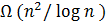for copy-only computation (Stoss, 1973). In the copy-only computation model the squares of the TM tapes contain symbols from an unknown set, with no operations among elements, so the TM heads can only copy the symbols. We show the same bound when we allow one operation, + between elements of the unknown set, where + has the properties of exclusive or (associative, commutative and idempotent). We also show a lower bound for oblivious Turing Machines, that is, TMs whose computation paths do not depend on the values of the input. We are able to reduce this case to the copy-only case.

Joint work with Janos Simon.

5:05-5:25      Despina Stasi (UIC), “Random Horn formulas and propagation connectivity for directed hypergraphs”

A definite Horn 3-CNF formula is a conjunction of Horn clauses of the form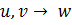. If the formula is viewed as a 3-uniform directed hypergraph, then the procedure of forward chaining in Horn formulas gives rise to a type of connectivity in the underlying directed hypergraph.

Let H be a random 3-uniform directed hypergraph selected by including each possible edge independently with probability p(n). We consider the property that almost every H is connected in this sense, and give upper and lower bounds for a threshold function.

5:25-5:45      Junghwan Shin (IIT), “Adversary Games in Secure/Reliable Network Routing”

In this paper, we consider security aspects of network routing in a game-theoretic framework where an attacker is empowered with a ability for intrusion into edges of the network; on the other hand, the goal of designer is to choose routing paths.

We interpret the secure routing problem as a two player zero sum game. The attacker can choose one or more edges for intrusion, while the designer has to choose paths between source-destination pairs for a given set of pairs. We give polynomial-time algorithms for finding mixed Nash equilibria if 1) the attacker is limited to a one-edge attack, 2) the designer has two source-destination pairs while the attacker is either limited to c edges, for given c, or the attacker incurs a cost for each edge attacked. Previous work gave an algorithm for one source-destination pair and multiple edge attacks.

5:45-6:05      Manolis Pountourakis (Northwestern University), “Group-strategy proof Cost-sharing mechanisms”

We study the problem of designing cost sharing mechanism for cost-sharing problems, where there is a set of agents interested in receiving a service. Each different subset of the players induces a possibly different cost. The players report their bids for getting serviced and the mechanism decides a set of players that are going to be serviced and how much each one of them is going to pay. We want our mechanisms to be group-strategyproof, i.e., no group of players has an incentive to bid untruthfully to increase the utility of at least one of its members while not decreasing the utilities of the rest. We determine conditions for the payment schemes that are necessary and sufficient for group-strategyproofness, which is the first complete characterization of this class of mechanisms.

Next, we study the implications of group-strategyproofness to our ability to construct budget balanced mechanisms. A mechanism is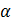-budget balanced if the sum of the payment is never greater than the cost of the service but also never less than anfraction of the cost. On the positive side, guided by this characterization we construct perfectly budget balanced mechanisms for the edge-cover game, whereas the previously known techniques to design group-stategyproof mechanisms could not achieve a budget balanced ratio greater than 1/2. Moreover, we provide a polynomial time algorithm for the allocation in this case, even though it is still open whether such an algorithm exists in general. On the negative side, we show that there exists a family of cost-functions where every group-strategyproof mechanism has arbitrarily poor budget balance. Finally, we show that there is no perfectly budget balance group-strategyproof mechanism for the set-cover game.

(Some of these results appeared in European Symposium of Algorithms 2010, under the title “A complete characterization of group-strategyproof mechanisms of cost-sharing”, Emmanouil Pountourakis and Angelina Vidali.)